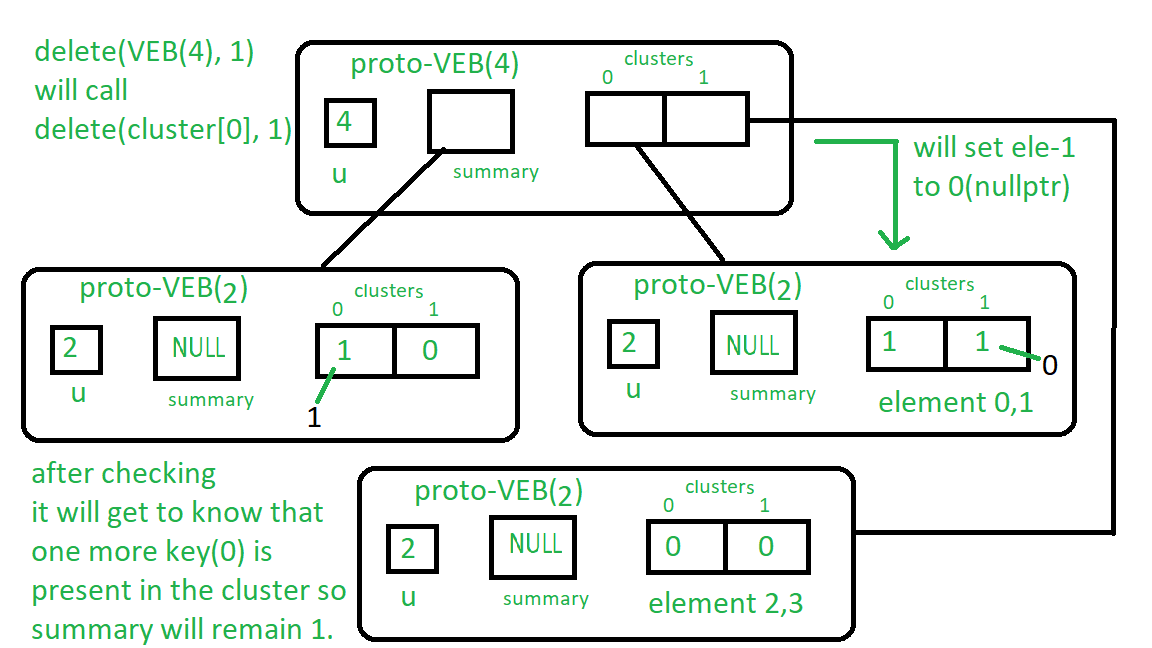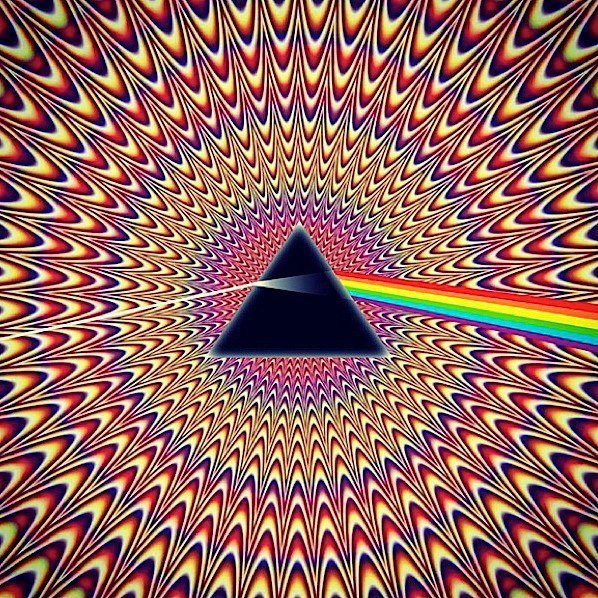# Proto Van Emde Boas Trees | Set 4 | Deletion

Please check previous sets of Proto Van Emde Boas Tree article first. It is highly recommended.

Procedure for delete:

1. Base Case: If we reach at Proto VEB with size 2 then we will check for whether the key is present or not if yes then we assign the pointer to nullptr which will set false to it presence.
2. Recursion:
• We recursively call delete function over the cluster of the keys i.e. high(key) and its position low(key).
• After we delete the key from the cluster (after we reach to the base case) we check whether there are any other keys are present in the cluster. If there is any key present then we can not set the summary to nullptr otherwise we will set the summary to nullptr by calling delete over summary.

Lets understand 1 delete on Proto-VEB of size 4:
First it will recursively call delete(cluster, 1).
So now the base case is satisfied so it will go at position 1 in the cluster Proto-VEB and will set it to nullptr if it is present.

Now we will check if any more keys are present in cluster (see the for loop in delete), 0 is present so delete(summary, 0) call is not going to execute and summary will remain same.

See the image below to understand it:

Follow the instructions written near the boxes from top to bottom.Below is the implementation:

 // C++ implementation of the approach  #include  using namespace std;     class Proto_Van_Emde_Boas {  public:      // Total number of keys      int universe_size;         // Summary      Proto_Van_Emde_Boas* summary;         // Clusters array of Proto-VEB pointers      vector clusters;         int root(int u)      {          return int(sqrt(u));      }         // Function to return cluster numbers      // in which key is present      int high(int x)      {          return x / root(universe_size);      }         // Function to return position of x in cluster      int low(int x)      {          return x % root(universe_size);      }         // Function to return the index from      // cluster number and position      int generate_index(int cluster, int position)      {          return cluster * root(universe_size) + position;      }         // Constructor      Proto_Van_Emde_Boas(int size)      {          universe_size = size;             // Base case          if (size <= 2) {                 // Set summary to nullptr as there is no              // more summary for size 2              summary = nullptr;                 // Vector of two pointers              // nullptr in starting              clusters = vector(size, nullptr);          }          else {                 // Assiging Proto-VEB(sqrt(u)) to summary              summary = new Proto_Van_Emde_Boas(root(size));                 // Creating array of Proto-VEB Tree pointers of size sqrt(u)              // first all nullptrs are going to assign              clusters = vector(root(size), nullptr);                 // Assigning Proto-VEB(sqrt(u)) to all its clusters              for (int i = 0; i < root(size); i++) {                  clusters[i] = new Proto_Van_Emde_Boas(root(size));              }          }      }  };     // Function that returns true if the  // key is present in the tree  bool isMember(Proto_Van_Emde_Boas* helper, int key)  {         // If key is greater then universe_size then      // returns false      if (key >= helper->universe_size)          return false;         // If we reach at base case      // the just return whether      // pointer is nullptr then false      // else return true      if (helper->universe_size == 2) {          return helper->clusters[key];      }      else {             // Recursively go deep into the          // level of Proto-VEB tree using its          // cluster index and its position          return isMember(helper->clusters[helper->high(key)],                          helper->low(key));      }  }     // Function to insert a key in the tree  void insert(Proto_Van_Emde_Boas*& helper, int key)  {      // If we reach at base case      // then assign Proto-VEB(1) in place      // of nullptr      if (helper->universe_size == 2) {          helper->clusters[key] = new Proto_Van_Emde_Boas(1);      }      else {             // Recursively using index of cluster and its          // position in cluster          insert(helper->clusters[helper->high(key)],                 helper->low(key));             // Also do the same recusion in summary VEB          insert(helper->summary, helper->high(key));      }  }     // Function to delete a key from the tree  void pveb_delete(Proto_Van_Emde_Boas*& helper, int key)  {         // Base case: If the key is present      // then make it nullptr      if (helper->universe_size == 2) {          if (helper->clusters[key]) {              delete helper->clusters[key];              helper->clusters[key] = nullptr;          }      }      else {             // Recursive delete to reach at the base case          pveb_delete(helper->clusters[helper->high(key)], helper->low(key));             bool isanyinCluster = false;             // Iterate over the cluster of keys to check whether          // any other key is present within that cluster          // If yes then we should not update summary to 0          // else update summary to 0          for (int i = helper->high(key) * helper->root(helper->universe_size);               i < (helper->high(key) + 1) * helper->root(helper->universe_size);               i++) {                 // If member is present then break the loop              if (isMember(helper->clusters[helper->high(key)], i)) {                  isanyinCluster = true;                  break;              }          }             // If no member is present then          // update summary to zero          if (isanyinCluster == false) {              pveb_delete(helper->summary, helper->high(key));          }      }  }     // Driver code  int main()  {      Proto_Van_Emde_Boas* hello = new Proto_Van_Emde_Boas(4);         cout << isMember(hello, 2);         insert(hello, 2);         insert(hello, 3);         cout << isMember(hello, 2);         pveb_delete(hello, 2);         cout << isMember(hello, 2);  }

Recurrence Relation for Delete:

T(u) = T(u) = 2T()) + O(log2())

Time Complexity : O(log2(u)*log2(log2(u)))

GeeksforGeeks has prepared a complete interview preparation course with premium videos, theory, practice problems, TA support and many more features. Please refer Placement 100 for details

My Personal Notes arrow_drop_upCheck out this Author's contributed articles.

If you like GeeksforGeeks and would like to contribute, you can also write an article using contribute.geeksforgeeks.org or mail your article to contribute@geeksforgeeks.org. See your article appearing on the GeeksforGeeks main page and help other Geeks.

Please Improve this article if you find anything incorrect by clicking on the "Improve Article" button below.

Improved By : shubham_singh